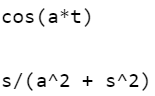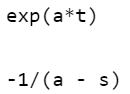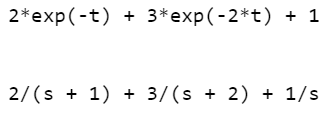# How to find inverse Laplace Transforms using MATLAB ?

In this article, we will see how to find Laplace Transform in MATLAB. Laplace Transform helps to simplify problems that involve Differential Equations into algebraic equations. Inverse Laplace Transform converts Laplace Domain Function F(s) into time-domain function f(t)Using the above function one can generate a Time-domain function of any Laplace expression.

Example 1: Find the Inverse Laplace Transform of## Matlab

 `% specify the variable a, t and s  ` `% as symbolic ones ` `syms a t s ` ` `  `% define function F(s)  ` `F = s/(a^2 + s^2); ` ` `  `% ilaplace command to transform into time ` `% domain function f(t)   ` `% Inverse Laplace Function ` `f1=ilaplace(F,s,t); ` ` `  `% Display the output value ` `disp(f1); ` ` `  `% Output can be verified by transforming  ` `% function f1 into Laplace Domain F(s) ` `f=laplace(f1,t,s);  ``% Laplace Function ` `disp(f);`

Output:which isExample 2: Find the Inverse Laplace Transform of 1/(s-a)

## Matlab

 `% specify the variable a, t and  ` `% s as symbolic ones ` `syms a t s ` ` `  `% define function F(s)  ` `F = 1/(s-a); ` ` `  `% ilaplace command to transform into ` `% time domain function f(t) ` `% Inverse Laplace Function ` `f1=ilaplace(F,s,t);  ` ` `  `% Display the output value ` `disp(f1); ` ` `  `% Output can be verified by transforming  ` `% function f1 into Laplace Domain F(s) ` `f=laplace(f1,t,s);  ``% Laplace Function ` `disp(f);`

Output:which isExample 3: Find the Inverse Laplace Transform of## Matlab

 `% specify the variable a, t and  ` `% s as symbolic ones ` `syms a t s ` ` `  `% define function F(s)  ` `F = 2/(s+1)+3/(s+2)+1/s; ` ` `  `% ilaplace command to transform into ` `% time domain function f(t)   ` `% Inverse Laplace Function ` `f1=ilaplace(F,s,t); ` ` `  `% Display the output value ` `disp(f1); ` ` `  `%Output can be verified by transforming  ` `% function f1 into Laplace Domain F(s) ` `f=laplace(f1,t,s);  ``% Laplace Function ` `disp(f);`

Output:which isWhether you're preparing for your first job interview or aiming to upskill in this ever-evolving tech landscape, GeeksforGeeks Courses are your key to success. We provide top-quality content at affordable prices, all geared towards accelerating your growth in a time-bound manner. Join the millions we've already empowered, and we're here to do the same for you. Don't miss out - check it out now!